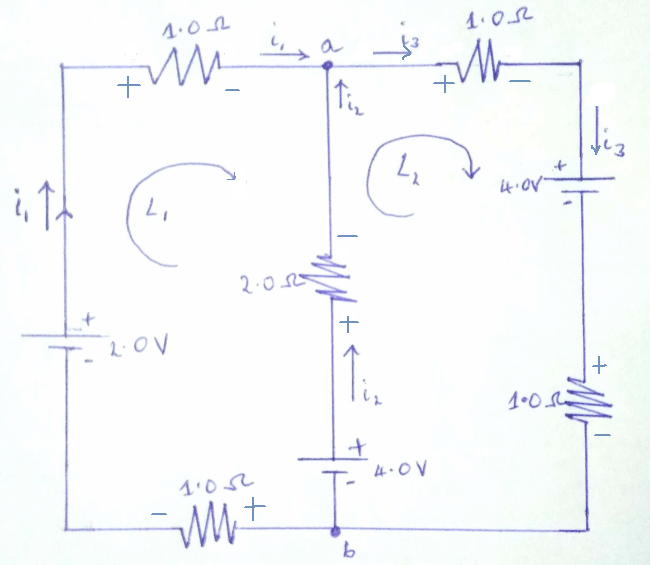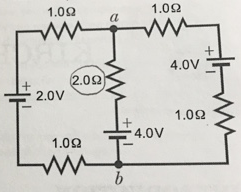# Problem: For the following circuit, you ultimately want to measure the power dissipation of the 2.0 Ω resistor:a) First, redraw the diagram on the page you are turning in.b) Define the directions of the currents arriving at the junction point a. Physically draw the directions of these currents on the diagram.c) Write an equation for the Kirchhoff Junction Rule for the current directions arriving at the junction point a as chosen in part a).d) Choose two Kirchhoff loops that include the 2.0 Ω resistor. Physically draw these two loops on the diagram. e) Write out a simple equation for each circuit element in your two Kirchhoff loops (note: you should have two equations). Clearly denote for each term whether it is a rise (+) in voltage or a drop (-) in voltage for your Kirchhoff loops. f) Combine your equations from part c) and part e) to solve for the current through the 2.0 Ω resistor.g) Use your result from part f) to solve for the power dissipation of the 2.0 Ω resistor.

###### FREE Expert Solution

(a)82% (142 ratings)###### Problem DetailsFor the following circuit, you ultimately want to measure the power dissipation of the 2.0 Ω resistor:

a) First, redraw the diagram on the page you are turning in.

b) Define the directions of the currents arriving at the junction point a. Physically draw the directions of these currents on the diagram.

c) Write an equation for the Kirchhoff Junction Rule for the current directions arriving at the junction point a as chosen in part a).

d) Choose two Kirchhoff loops that include the 2.0 Ω resistor. Physically draw these two loops on the diagram.

e) Write out a simple equation for each circuit element in your two Kirchhoff loops (note: you should have two equations). Clearly denote for each term whether it is a rise (+) in voltage or a drop (-) in voltage for your Kirchhoff loops.

f) Combine your equations from part c) and part e) to solve for the current through the 2.0 Ω resistor.

g) Use your result from part f) to solve for the power dissipation of the 2.0 Ω resistor.